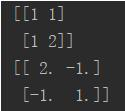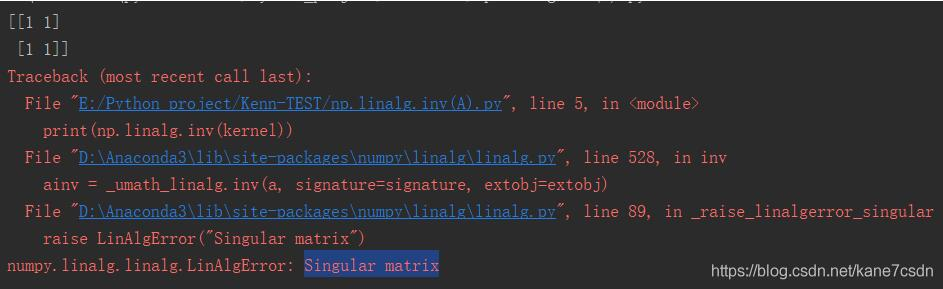• 2022-05-06 00:10:53

4X4矩阵求逆：

import numpy as np
A = np.mat("1 2 3 0; 4 5 6 0; 9 8 10 0;0 0 0 1")
print("A\n", A)
inverse = np.linalg.inv(A)
print("inverse: \n", inverse)

更多相关内容
• 可用于求解希尔密码密钥的逆矩阵，根据逆矩阵与密文相乘再mod 26计算出明文
• python求矩阵逆、伪逆、转置、矩阵乘法

# 1. python求矩阵的转置

G1 = np.transpose(G)

>>> import numpy as np
>>> G = np.array([[1,0,0,1],[0,1,0,-1],[0,0,1,1]])
>>> G1=np.transpose(G)
>>> G1
array([[ 1,  0,  0],
[ 0,  1,  0],
[ 0,  0,  1],
[ 1, -1,  1]])

# 2. python求矩阵乘法

G2 = np.dot(G,G1)

>>> import numpy as np
>>> G = np.array([[1,0,0,1],[0,1,0,-1],[0,0,1,1]])
>>> G1=np.transpose(G)
>>> G1
array([[ 1,  0,  0],
[ 0,  1,  0],
[ 0,  0,  1],
[ 1, -1,  1]])
>>> G2 = np.dot(G,G1)
>>> G2
array([[ 2, -1,  1],
[-1,  2, -1],
[ 1, -1,  2]])

# 3. python求矩阵的逆

G3 = np.linalg.inv(G2)

>>> import numpy as np
>>> G = np.array([[1,0,0,1],[0,1,0,-1],[0,0,1,1]])
>>> G1=np.transpose(G)
>>> G1
array([[ 1,  0,  0],
[ 0,  1,  0],
[ 0,  0,  1],
[ 1, -1,  1]])
>>> G2 = np.dot(G,G1)
>>> G2
array([[ 2, -1,  1],
[-1,  2, -1],
[ 1, -1,  2]])
>>> G3 = np.linalg.inv(G2)
>>> G3
array([[ 0.75,  0.25, -0.25],
[ 0.25,  0.75,  0.25],
[-0.25,  0.25,  0.75]])

# 4. python求矩阵的伪逆

K = np.linalg.pinv(J)

>>> import numpy as np
>>> J = np.array([[1,0,0,1],[1,1,0,0],[0,1,1,0],[0,0,1,1]])
>>> K = np.linalg.pinv(J)
>>> K
array([[ 0.375,  0.375, -0.125, -0.125],
[-0.125,  0.375,  0.375, -0.125],
[-0.125, -0.125,  0.375,  0.375],
[ 0.375, -0.125, -0.125,  0.375]])

# 5 python求解矩阵的特征值

B = np.linalg.eigvals(A)

>>> A = np.mat("0 0 0; -1 1 -1; 1 -1 1")
>>> A
matrix([[ 0,  0,  0],
[-1,  1, -1],
[ 1, -1,  1]])
>>> B = np.linalg.eigvals(A)
>>> B
array([2., 0., 0.])

# 6 python求解矩阵的特征值和特征向量

B,C = np.linalg.eig(A)

>>> A = np.array([[1,0,-1,0],[0,1,0,-1],[-1,0,1,0],[0,-1,0,1]])
>>> A
array([[ 1,  0, -1,  0],
[ 0,  1,  0, -1],
[-1,  0,  1,  0],
[ 0, -1,  0,  1]])
>>> B,C = np.linalg.eig(A)
>>> B
array([2., 0., 2., 0.])
>>> C
array([[ 0.70710678,  0.70710678,  0.        ,  0.        ],
[ 0.        ,  0.        ,  0.70710678,  0.70710678],
[-0.70710678,  0.70710678,  0.        ,  0.        ],
[ 0.        ,  0.        , -0.70710678,  0.70710678]])

注： 这是返回单位化的特征向量

展开全文• 我就废话不多说了，大家还是直接看代码吧~import numpy as npkernel = np.array([1, 1, 1, 2]).reshape((2, 2))print(kernel)print(np.linalg.inv(kernel))注意，Singular matrix奇异矩阵不可求逆补充：python+numpy...

我就废话不多说了，大家还是直接看代码吧~

import numpy as np

kernel = np.array([1, 1, 1, 2]).reshape((2, 2))

print(kernel)

print(np.linalg.inv(kernel))注意，Singular matrix奇异矩阵不可求逆补充：python+numpy中矩阵的逆和伪逆的区别

定义：

对于矩阵A，如果存在一个矩阵B，使得AB=BA=E，其中E为与A,B同维数的单位阵，就称A为可逆矩阵(或者称A可逆)，并称B是A的逆矩阵，简称逆阵。(此时的逆称为凯利逆)

矩阵A可逆的充分必要条件是|A|≠0。

伪逆矩阵是逆矩阵的广义形式。由于奇异矩阵或非方阵的矩阵不存在逆矩阵，但可以用函数pinv(A)求其伪逆矩阵。

基本语法为X=pinv(A),X=pinv(A,tol),其中tol为误差,pinv为pseudo-inverse的缩写：max(size(A))*norm(A)*eps。

函数返回一个与A的转置矩阵A' 同型的矩阵X，并且满足：AXA=A,XAX=X.此时，称矩阵X为矩阵A的伪逆，也称为广义逆矩阵。

pinv(A)具有inv(A)的部分特性，但不与inv(A)完全等同。

如果A为非奇异方阵，pinv(A)=inv(A)，但却会耗费大量的计算时间，相比较而言，inv(A)花费更少的时间。

代码如下：

1.矩阵求逆

import numpy as np

a = np.array([[1, 2], [3, 4]]) # 初始化一个非奇异矩阵(数组)

print(np.linalg.inv(a)) # 对应于MATLAB中 inv() 函数

# 矩阵对象可以通过 .I 求逆,但必须先使用matirx转化

A = np.matrix(a)

print(A.I)

2.矩阵求伪逆

import numpy as np

# 定义一个奇异阵 A

A = np.zeros((4, 4))

A[0, -1] = 1

A[-1, 0] = -1

A = np.matrix(A)

print(A)

# print(A.I) 将报错，矩阵 A 为奇异矩阵，不可逆

print(np.linalg.pinv(A)) # 求矩阵 A 的伪逆(广义逆矩阵)，对应于MATLAB中 pinv() 函数

这就是矩阵的逆和伪逆的区别

截至2020/10/4，matrix函数还可以使用，但已经过时，应该是mat函数这种。

以上为个人经验，希望能给大家一个参考，也希望大家多多支持我们。如有错误或未考虑完全的地方，望不吝赐教。

时间： 2021-03-09

展开全文• import numpy as np A = np.matrix([[1,2,3],[4,5,6],[7,8,9]]) B = np.array([1,2,3,4,5,6,7,8,9]).reshape((3,3)) print(A) print(B) print("*" * 50) print(np.linalg.inv(A)) print(np.linalg.inv(B)) ...
import numpy as np

A = np.matrix([[1,2,3],[4,5,6],[7,8,9]])
B = np.array([1,2,3,4,5,6,7,8,9]).reshape((3,3))
print(A)
print(B)
print("*" * 50)
print(np.linalg.inv(A))
print(np.linalg.inv(B))


结果为：

[[1 2 3]
[4 5 6]
[7 8 9]]
[[1 2 3]
[4 5 6]
[7 8 9]]
**************************************************
[[ 3.15251974e+15 -6.30503948e+15  3.15251974e+15]
[-6.30503948e+15  1.26100790e+16 -6.30503948e+15]
[ 3.15251974e+15 -6.30503948e+15  3.15251974e+15]]
[[ 3.15251974e+15 -6.30503948e+15  3.15251974e+15]
[-6.30503948e+15  1.26100790e+16 -6.30503948e+15]
[ 3.15251974e+15 -6.30503948e+15  3.15251974e+15]]

展开全文• 方法1： from numpy import * import numpy as np A=np.array([[1,2],[3,4]]) B=np.linalg.inv(A) print(B) print(np.dot(B,A)) 方法2： from numpy import * ...print(a2*a1)
• 假定有方阵A， A 的逆矩阵Python 中使用 numpy 库函数命令实现。 import numpy as np A_1 = np.linalg.inv(A) # 逆矩阵（奇异矩阵没有逆矩阵，不可以用此命令计算） A_2 = np.linalg.pinv(A) # 伪逆矩阵...开发语言 后端
• 今天小编就为大家分享一篇python/sympy求解矩阵方程的方法，具有很好的参考价值，希望对大家有所帮助。一起跟随小编过来看看吧
• 如下所示： def save(data, path): f = xlwt.Workbook() # 创建工作簿 sheet1 = f.add_sheet(u'sheet1', cell_overwrite_ok=True) # 创建sheet ...以上这篇Python 保存矩阵为Excel的实现方法就是小编分
• 今天小编就为大家分享一篇对Python矩阵或者数组相减的法则详解，具有很好的参考价值，希望对大家有所帮助。一起跟随小编过来看看吧
• 本文实例为大家分享了python实现矩阵打印的具体代码，供大家参考，具体内容如下 之前面试嵌入式软件的一道题，用c实现矩阵打印，考场上并没有写出来，之后总感觉自己写不出来也就没有去实现，在网上找也没能找到答案...
• Python中，无论是方阵的逆矩阵，还是非方阵的伪逆矩阵，都有现成的模块可供调用
• A的等于U的乘于L的，L的就利用下三角矩阵求逆算法进行求解，U的可以这样：先将U转置成下三角矩阵，再像对L求逆一样对U的转置求逆，再将得到的结果转置过来，得到的就是U的。因此，关键是下三角矩阵的...
• python进行矩阵运算的方法： 1、矩阵相乘 >>>a1=mat([1,2]); >>>a2=mat([,]); >>>a3=a1*a2 #1*2的矩阵乘以2*1的矩阵，得到1*1的矩阵 >>> a3 matrix([]) 2、矩阵对应元素相乘 >>>a1=mat([1,1]); >>>a2=mat...
• 稀疏矩阵是机器学习中的重要工具。本代码为自己编写。初学者，希望大家批评指正。
• 本文介绍了纯python进行矩阵的相乘运算的方法示例，分享给大家，具体如下： def matrixMultiply(A, B): # 获取A的行数和列数 A_row, A_col = shape(A) # 获取B的行数和列数 B_row, B_col = shape(B) # 不能...
• 今天小编就为大家分享一篇关于Python最小二乘法矩阵，小编觉得内容挺不错的，现在分享给大家，具有很好的参考价值，需要的朋友一起跟随小编来看看吧
• 1、用Python求解矩阵的秩 import numpy as np a = np.array([[1, 2, 3], [3, 5, 6], [1, 3, 5]]) ...2、用Python求解矩阵逆矩阵 # 求逆矩阵 print(np.linalg.inv(a)) 结果： array([[ 7., -1., -3.], [-numpy 人工智能 机器学习
• 本文采用了以下方法进行值：矩阵计算法、定义法、分治法和Strassen方法。这里我们使用Matlab以及Python对这个问题进行处理，比较两种语言在一样的条件下，运算速度的差别。 编程语言 Python 具体代码 #-*- coding...
• python矩阵求逆及矩阵相乘，求解，和矩阵转化等有关矩阵算法
• 您可能感兴趣的文章:python+numpy实现的基本矩阵操作示例numpy实现合并多维矩阵、list的扩展方法python生成1行四列全2矩阵的方法Python 使用Numpy对矩阵进行转置的方法Python+numpy实现矩阵的行列扩展方式
• 今天小编就为大家分享一篇Python根据欧拉角旋转矩阵的实例，具有很好的参考价值，希望对大家有所帮助。一起跟随小编过来看看吧
• 矩阵A第i行第j列元素的余矩阵 p=len(A)#矩阵的行数 q=len(A)#矩阵的列数 C=[[A[x][y] for y in ... #按第一行展开递归求矩阵的行列式 p=len(A)#矩阵的行数 q=len(A)#矩阵的列数 if(p==1 and q==1): retur
• 输入一个正整数N（N不大于100），输出一个n行的蛇形矩阵。 示例输入： 5 示例输出： 1 3 6 10 15 2 5 9 14 4 8 13 7 12 11 解法一： while True: try: N = int(input()) tmp_begin = 1 # 第一行的数 for i in ...
• #模26运算下，求矩阵。 #使用伴随矩阵求逆矩阵法。 #整个精度保持在整数位（方便模运算）。 import numpy as np list_A1 = [] #目标矩阵A1 A1 = np.array([[3,13,21,9],[15,10,6,25],[10,17,4,8],[1,23,7,...numpy...